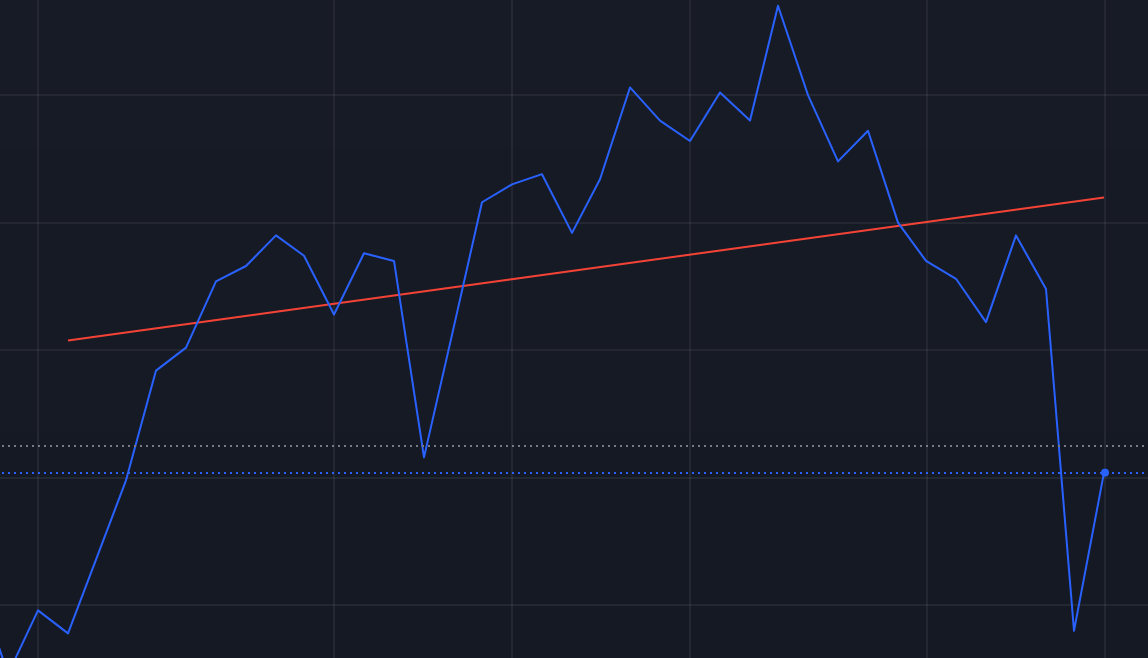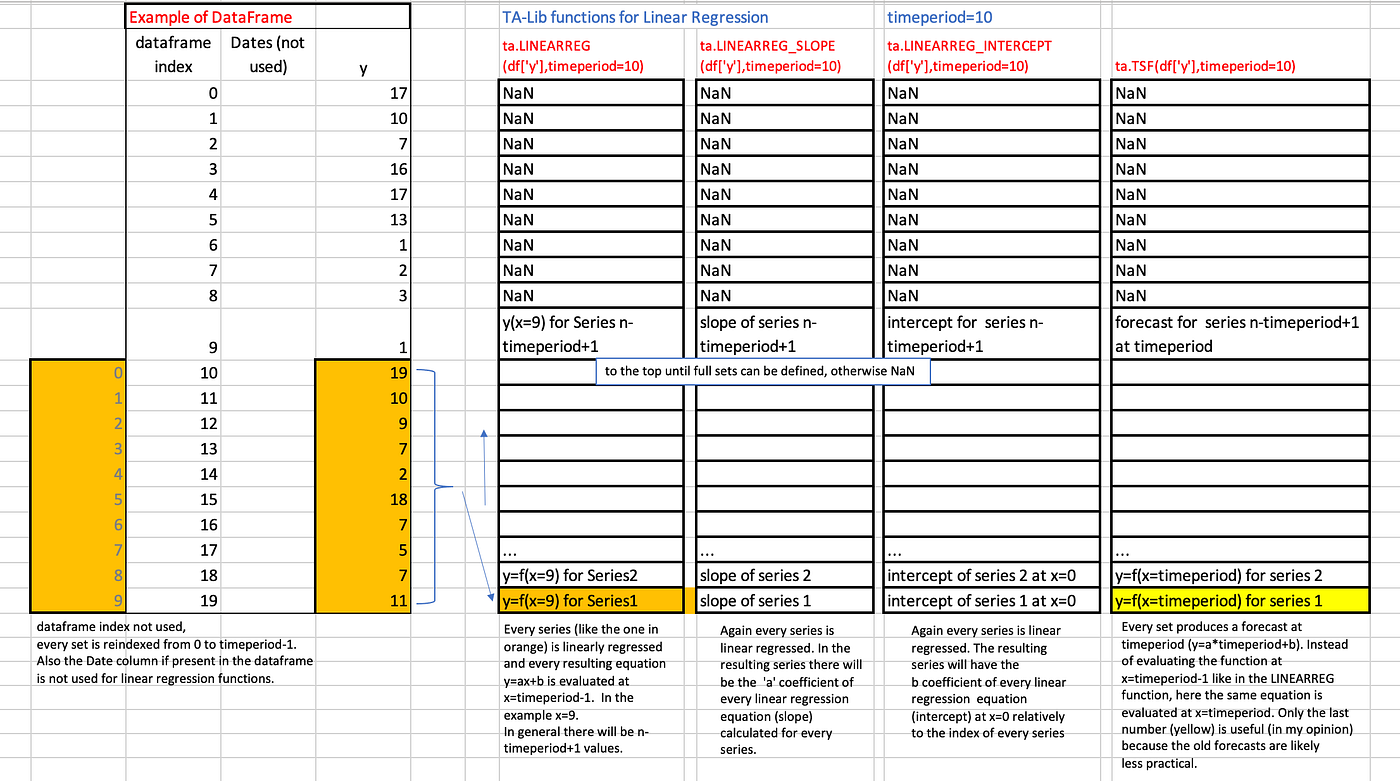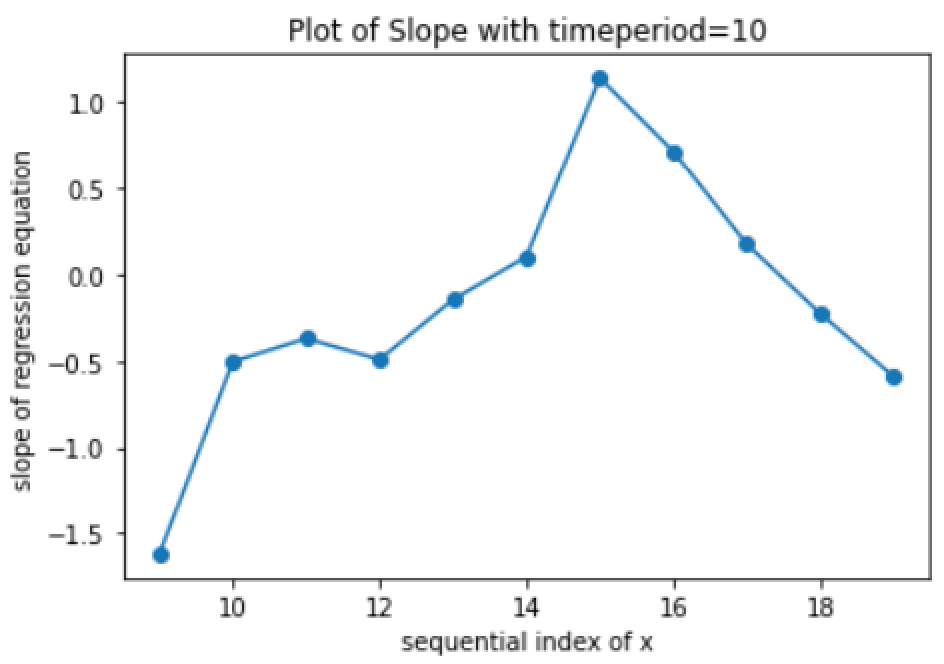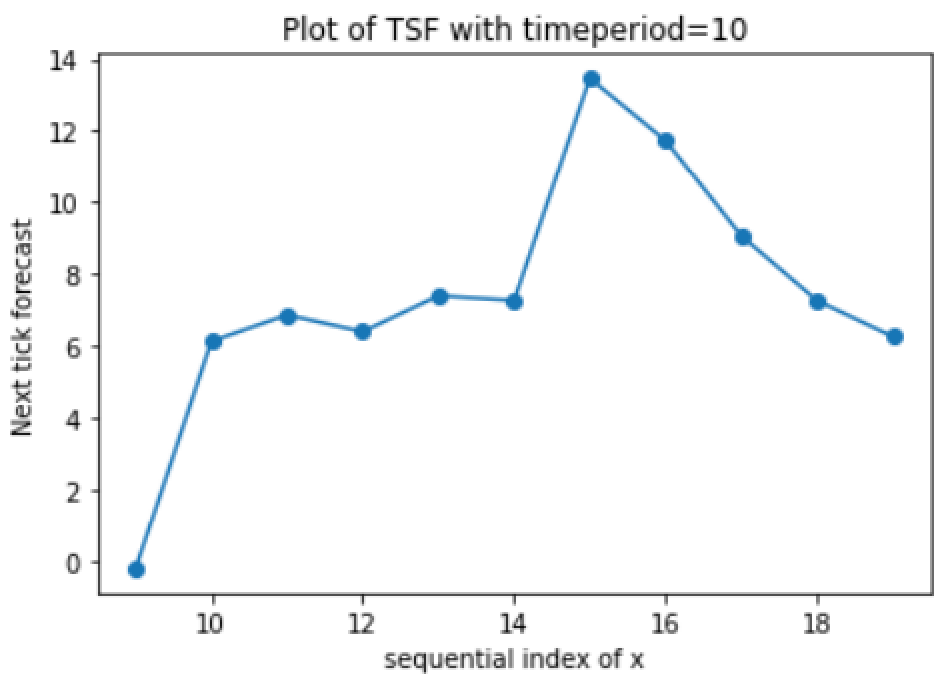# Linear Regression with TA-LibThe Linear Regression functions within TA-Lib are a simplification of others Linear Regression functions available through other Python Libraries. Nevertheless it could be very useful in the context of Technical Analysis of stock market indicators (Source: Image of the author)

# ta.LINEARREG(series of values,timeperiod)An example of a sample dataframe on the left and the results of the functions on the right. (Source: Image of the author)

# ta.LINEARREG_ANGLE (column with y values, timeperiod)Quick and dirty plot of the new series created with tje ta.LINEARREG_SLOPE function. Every point is the slope evaluated with the regression equation over all of timeperiod=10 sets of values, at x=9. (Source: Image of the author)

# ta.LINEARREG_TSF (column with y values, timeperiod)Quick and dirty plot of the new series created with ta.TSF function. Every point is the next tick(*) forecast evaluated with the regression equation over all of timeperiod=10 sets of values, at x=10. (*) tick can whatever you have in the dataframe: days, months, years, minutes… As you can see the shape of the plot is similar to the previous plot but changes the units of measures along the y axis (Source: Image of the author)